# The Time-Evolution Operator

Given a wave function, at a time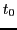, the instantaneous change can be found from the Time-Evolution operator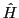, also known as the Hamiltonian;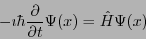(1)

In principle, this equation can be integrated to give the value offor later times.

The non-relativistic Hamiltonian consists of a kinetic part, which is simply the differential operator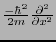, and a potential part which is a function of x. The simplest case is the ''free particle, in which the potential is constant, independent of x.

Here is a video of the time evolution of a momentum eigenstate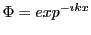;

The time-evolution of a momentum eigenstate consists of nothing more than a steady rotation of the phase. As you can see from the absolute magnitude displayed in the lower half of the frame, this phase rotation does not alter the position of the particle. It is equally likely to be anywhere in the coordinate space, and that fact is independent of time.

Jerome Berryhill 2013-05-09